5/29/2020 11:41:56 AM Matej Petrinović, Mario PilijaThe flow of every project in a perfect world goes approximately thus: there are a lot of tasks at the beginning, so we conduct a plan how to deal with them; we start to solve them and the number of tasks decreases over time. Eventually, of course, there are no tasks left and the project is finished. However, in reality, every now and then an unexpected or unforeseen task comes up and slows down the process a bit.

What if we could predict those situations, take them into consideration in the planning phase of an iteration and by doing so, significantly save time and money?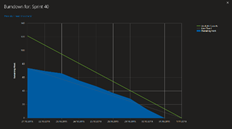Picture 1

If you’re into modern project planning, you’re probably “trying” to practice Agile methods. Before the beginning of an iteration everything is well groomed, the work plan for the iteration is a piece of cake and the team is super excited to get their hands dirty. However, initial excitement ends very soon. Development team finds things that are more complicated than they predicted, unplanned work is accumulating, team leaders are pulling their hair out and aging 5 years per minute.

Sounds familiar?

What if we could predict those situations and take them into consideration in the planning phase of an iteration and reduce the risk by doing that?

## Let’s dig deeper…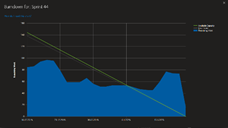Picture 2

The flow of every project in a perfect world goes approximately thus: there are a lot of tasks at the beginning, so we conduct a plan how to deal with them; we start to solve them and the number of tasks decreases over time. Eventually, of course, there are no tasks left and the project is finished. However, in reality, every now and then an unexpected or unforeseen task comes up and slows down the process a bit.

That’s why we’ve conducted this analysis for a start as a part of regular retrospective activities, but very soon we’ve concluded that it would be nice to foresee this for future iterations or future time period. This knowledge should be used then as a risk management factor and one of the KPI’s for measuring team success over time.

For instance, let’s say that we have data about a past iteration in the following format:

 IterationSK Iteration Name Iteration Path Start Date Finish Date abc Sprint XY \Project_Name\Phase 9\Sprint XY 2019-02-04 2019-02-15

For this iteration, we can easily select open tasks on a particular date:

 Sprint Week 1 04/02/2019 05/02/2019 06/02/2019 07/02/2019 08/02/2019 Open task number 15 16 8 15 8 Sprint Week 2 11/02/2019 12/02/2019 13/02/2019 14/02/2019 15/02/2019 Open task number 14 16 8 22 10

How does that look like over time? Let's take a look at the following graph: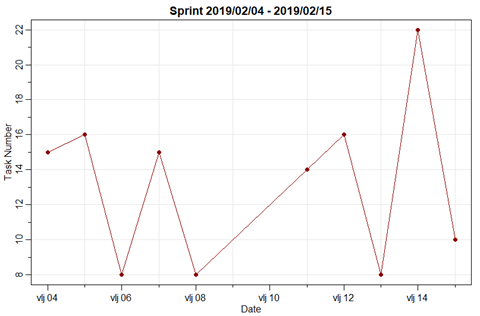Picture 3

In theory, from the beginning to the end of iteration the number of tasks should decrease. That's why we should set apart those tasks that stand out and regard them as anomaly, i.e. outlier in a time series.

Theoretically, it should look something like this: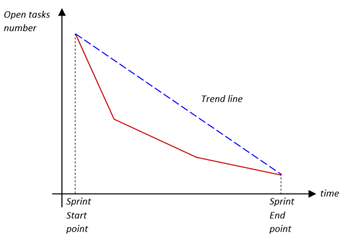Picture 4

Because a sprint takes two weeks, i.e. 10 dates, we can apply neither statistical nor machine learning algorithms.  So, we have to be creative and think a little bit outside of the box.  Let’s apply a very simple method. We need to collect all the peaks in time series data. Because of the small number of data, we can simply iterate through time series and do some subtractions and comparisons.  If we look what a peak is, we can simply apply if-else statement in anomaly search procedure, such as this algorithm:

##### Anomaly Algorithm (time_series):
1. Input time series data
2. Make empty anomaly vector A
3. Set first element of A to some negative number (eg. -1)
4. Check conditions and do the following:
•  ifyi-1 < yi and yi+1 < yappend A with yi
• else: append A with some negative number (eg. -1)
5. Set the last element of A to some negative number (eg. -1)
6. Get the indexes of positive element of A
7. Take the subset of first data frame to get number of tasks and date which are considered anomalies

After applying the previous algorithm, we get the following table:

 Time index Date Open tasks number 2 05/02/2019 16 4 07/02/2019 15 7 12/02/2019 16 9 14/02/2019 22

Here we can see some of the tasks which were conducted during the time period which has, in this analysis, turned out to be an anomaly:

Quite a lot of them, for sure!

Before we get started, let’s remember the famous saying of George E. P. Box, a British statistician: All models are wrong, but some are useful”.

Our next challenge will be to predict the number of future anomalies for future sprints. For further analysis we’ll use R programming language for statistical analysis.

## Anomalies prediction based on past anomalies count

As we’ve done before, we will do this for every sprint. For each sprint we’ll calculate the number of anomalies and form the time series of sprint anomalies. With that time series we’ll be able to create a mathematical model for prediction of a future anomaly number.
How to create such time series? Look at the picture: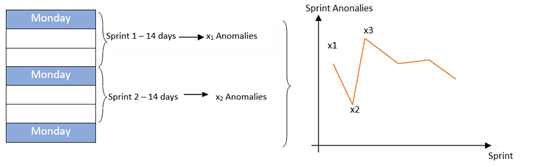Picture 5

After following steps from the previous picture, we get the following graph: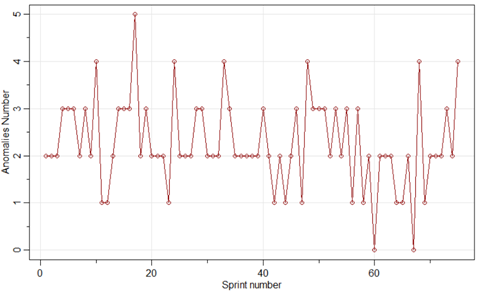Picture 6

From the picture we can see that the number of anomalies varies from zero to five.  Since we have 75 sprints (from 2017 to now), we can make a model for prediction. We have taken a project that we’ve been working on for 3 years because the bigger the size of data, the better the model, and the predictions are more precise. For forecasting we’ll use an ARIMA (p,d,q) model, see , where p is the order of the ‘Auto Regressive’ (AR) term, q is the order of the ‘Moving Average’ (MA) term and d is a number of differencing . After making an ARIMA (3,0,2) model, we can get a forecast for the number of anomalies in the future sprints. Let’s take, e.g., h = 15 future sprints, and look at an anomaly's forecasting on the following graph: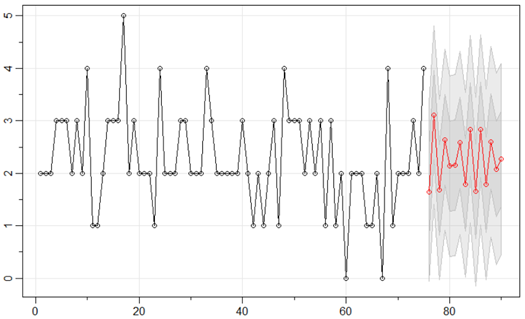Picture 7

From the picture it can be seen that in the next sprint almost 2 anomalies are predicted, then 3 anomalies, and so on. We’ve calculated RMPSE (root mean prediction squared error, see ) as: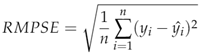Root mean prediction squared error is a statistical metric that tells us how well a model preforms on test data. It’s a simply calculated sum of squared differences of real and predicted values. Then we divide this with the number of test data and finally take a square root. RMPSE for this model is 0.8559363, which is low. The lower the better.

What if we look at the remaining work hours through every sprint and try classifying anomalies on that sprint and try to do a prediction? Ok, let’s look at the remaining work hours graph for one sprint: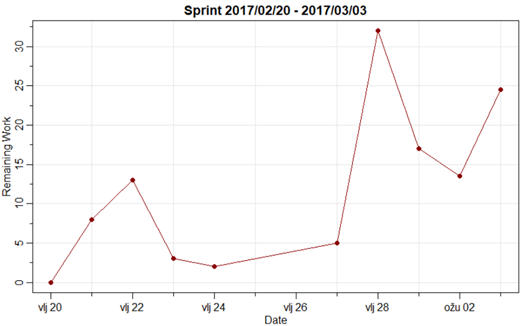Picture 8

Here we can also apply our Anomaly Algorithm to get dates of anomalies and try predicting for next sprint(s). Let’s do the previous. Get the number of anomalies for every sprint and form time series of Anomalies over Sprints. How does it look? Here’s the graph: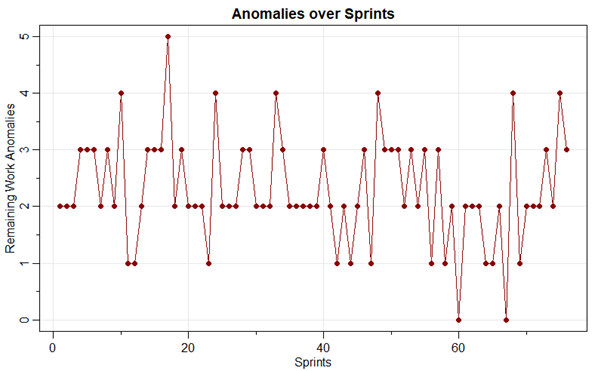Picture 9

Looks familiar? Are you surprised? Don’t be. It’s logical. That’s happened because the open tasks number and remaining work are in correlation or cointegration. In(de)creasing one affects in(de)creasing of another. So, if you want to predict the number of anomalies, it does not matter which time series you are using, you’ll get the same results.

However, take this prediction with a grain of salt, because predictions are nothing but theoretical. But they can give you some idea of a possible future scenario.

## Conclusion

What can we do with all those findings about anomalies? We can use this as a part of a Sprint retrospective analysis and track it over time as one of the team's goals to reduce it. We can use it as a risk management factor in planning or even as one of the team's success measurements.

Where do we go from here? Maybe to integrate the model into a PowerBI reporting system? Maybe to integrate it into a Risk management application? Only the sky is the limit… 😊

References:
 https://en.wikipedia.org/wiki/Mean_squared_error
 https://www.machinelearningplus.com/time-series/arima-model-time-series-forecasting-python/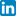Share